# Martin Orr's Blog

## A brief account of my research

Posted by Martin Orr on Thursday, 20 September 2012 at 17:39

I recently had to write an account of my research for a non-specialist. I think I took it more seriously than necessary -- I am not sure that anyone will read it. But it might interest some readers of this blog, so I shall put it here. I have just put the paper containing this work on Arxiv.

## Main steps of the proof of the period theorem

Posted by Martin Orr on Friday, 03 August 2012 at 11:06

Today I will explain how to prove the Masser-Wüstholz Period Theorem starting from the Key Proposition, an weaker existence result for abelian subvarieties of bounded degrees. The Key Proposition and the Tangent Space Lemma, which I mention briefly, are the main results proved by Masser and Wüstholz by the techniques of transcendental number theory on their way to the Period Theorem.

Recall that the Period Theorem is a bound for the degree of the smallest abelian subvariety of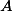whose tangent space contains a given period. In the Key Proposition we find a subvariety of bounded degree whose tangent space satisfies the very weak condition of being inside a chosen hyperplane in. In particularneed not be in the tangent space of the subvariety. However we use the Key Proposition and induction to prove the Strong Proposition, which gives a subvariety whose tangent space does contain. Several applications of the Strong Proposition with different hyperplanes inprove the Period Theorem.

## The matrix lemma for elliptic curves

Posted by Martin Orr on Friday, 25 May 2012 at 14:00

Letbe a principally polarised abelian variety of dimensionover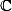. We can associate withacomplex matrix called the period matrix which roughly speaking describes a basis for the image of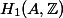in(actually it is not really the period matrix as it is only defined up to the action ofon the Siegel upper half space; we can make it nearly unique by forcing it to be in a particular fundamental domain).

The matrix lemma says that, ifis defined over a number field, then the entries of the imaginary part of the period matrix cannot be too large with respect to the height of(Faltings height or modular height).

Matrix lemma. (Masser 1987) Letbe a principally polarised abelian variety of dimensionover a number field. Letbe the period matrix forin the standard fundamental domain of the Siegel upper half space. There is a constantdepending only onsuch that all the entries ofsatisfy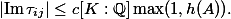Last time I used a lower bound for the lengths of non-zero periods in the proof of the isogeny theorem. This follows from the matrix lemma as we can easily relate lengths of periods and the period matrix.

Today I will prove the matrix lemma for elliptic curves. The general proof requires various facts about Siegel modular forms and also uses a funny choice of level structure due to Igusa (I do not understand why). But the basic structure of the proof is already visible in the elliptic curves case and we can be concrete about the modular forms involved, using only facts I learned in Part III.

## The Masser-Wüstholz isogeny theorem

Posted by Martin Orr on Wednesday, 25 April 2012 at 14:09

Letand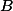be two isogenous abelian varieties over a number field. Can we be sure that there is an isogeny between them of small degree, where "small" is an explicit function ofand? In particular, our bound should not depend on; this means that the bound will imply Finiteness Theorem I, and hence the Shafarevich, Tate and Mordell conjectures.

The Masser-Wüstholz isogeny theorem answers this question, at least subject to a minor condition on polarisations (I think that this was removed in a later paper of Masser and Wüstholz but it is not too important anyway -- when deducing Finiteness Theorem I you can remove the polarisation issue with Zarhin's Trick).

Theorem. (Masser, Wüstholz 1993) Letandbe principally polarised abelian varieties over a number field. Suppose that there exists some isogeny. Then there is an isogenyof degree at most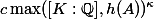whereandare constants depending only on the dimension of.

We will prove this using the Masser-Wüstholz period theorem which I discussed last time.

## The Masser-Wüstholz period theorem

Posted by Martin Orr on Friday, 30 March 2012 at 12:20

I wanted to write a post about the Masser-Wüstholz isogeny theorem, which gives a quantitative version of Finiteness Theorem I. But it turned out to be too long so for today I will focus on the main ingredient in the proof of the isogeny theorem: the Masser-Wüstholz period theorem.

The period theorem gives a bound for the degree of the smallest abelian subvariety of a fixed abelian varietyhaving a given period ofin its tangent space. In this post I will explain the statement of the period theorem, in particular defining the degree of a (polarised) abelian variety, and give some properties of the degree which will be used in the proof of the isogeny theorem.

Period Theorem. (Masser, Wüstholz 1993) Letbe an abelian variety defined over a number fieldwith a principal polarisation. For any non-zero periodof, the smallest abelian subvarietyofwhose tangent space containssatisfieswhereandare constants depending only on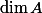.

Masser and Wüstholz gave a value forofwhere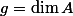. For myself, I am only interested in the existence of such a bound, but work has been done on improving it. If I correctly understand a recent preprint of Gaudron and Rémond, they show that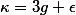suffices.